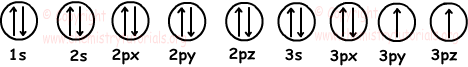## Atomic Structure Exam3 and Problem Solutions

Atomic Structure Exam3 and  Problem Solutions

1. Is there spherical symmetry in 6C atom?

Solution:

Electron configuration of C;

C: 1s22s22p2

Since p orbital includes 2 electrons there is no spherical symmetry in this element. To have spherical symmetry elements must have filled or half filled orbitals.

2. White phosphorus and red phosphorus are allotrope of phosphorus element. Which ones of the following statements are true for them;

I. Electron structures of atoms of them are different

II. They have different densities

III. Chemical properties of compound P2O5 formed by these allotrope are different.

Solution:

I. Allotrope include atoms belong to same element. Thus, electron structures of atoms must be same. I is false

II. Since allotrope have different physical properties, densities of them must be different. II is true.

III. Chemical properties of allotrope with other elements are same. III is false.

3. Which ones of the following couples have same chemical properties? (11H, 12D)

I. 2656Fe+2 and 2656Fe+3

II. 2656Fe and 2756Co

III. H2O and D2O

Solution:

To have same chemical properties, matters must have same number of protons and electrons.

I. Since they have equal number of protons but different number of electrons, their chemical properties are different.

II. Both number of protons and neutrons are different. So, chemical properties of them are also different.

III. They have same numbers of proton and electron. They have same chemical properties.

4. Which ones of the following statements are false for X and Y atoms;

X: 1s22s22p63s1

Y: 1s22s22p63s23p64s1

I. Y is excited state of X

II. X and Y are atoms of same element

III. Y is more stable than X

Solution:

I. Electron configuration of X is written according to ground state of atom. But, in Y one electron of 3s is written to 4s orbital. Thus,Y is excited state of X. If energy is given to X, it can turn into Y. I is true.

II. Since they both have 11 electrons they belong to same element. II is true.

III. Since energy of X is smaller than energy of Y, it is more stable than Y. III is false.

5. If number of filled orbitals of X is 7 and half filled orbitals is 2, which ones of the followings can be found?

I. Atomic number

II. Number of valence electrons

III. Mass number

Solution:

We draw 7 filled and 2 half filled orbitals like;Electron configuration :

1s22s22p63s23p4

Number of electrons: 2+2+6+2+4=16

In neutral atom, number of electrons is equal to number of protons and atomic number. So, using given data we can find atomic number of element. We can also find valence electrons of element by adding them.

3p and 3s are valence orbitals.

Number of valence electrons=2+4=6

We have to know number of neutrons to find mass number. From given data we can not find mass number.

6. Which of the following statements are true for 17X, 20Y and 18Z elements?

I. Y and X elements form YX2 compound

II. Y and Z+1 ion are isoelectronic

III. Electron configuration of Z+1 last with 4s2 orbital.

Solution:

17X has electron configuration: 1s22s22p63s23p5

20Y has electron configuration: 1s22s22p63s23p64s2

X accepts one electron and Y gives two electrons to have noble gas electron configuration. X is nonmetal and Y is metal, they form following compound;

YX2 I is true

Since electron configuration of Y and Z+1 are different they are nor isoelectronic. II is false.

Z+1 has electron configuration: 1s22s22p63s23p5

III is false

Related